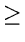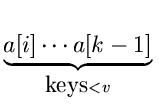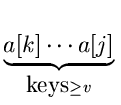Next:9.6.2 Algorithm for PartitioningUp:9.6 Quick SortPrevious:9.6 Quick Sort

## 9.6.1 Algorithm:

To sort the records a[i], a[i + 1],..., a[j], in place.
quicksort (i, j)

{

if (a[i] ... a[j] contain at least two distinct keys)

{

let v be the larger of the first two distinct keys;

Partition a[i] ... a[j] so that for some k between i + 1 and j,

a[i] ... a[k - 1] all have keys <v, and

a[k] ... a[j] all have keysv;

quicksort (i, k - 1);

quicksort (k, j);

}

}v is called the pivot. It could be any element such that the resulting partition desirably has two equal sized groups.

eEL,CSA_Dept,IISc,Bangalore